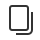• 介绍
• 设计
• 开发
• 数据
• 运营
• 第三方平台
• 社区# 语句

## # if 语句

• `if (expression) statement`： 当 `expression``truthy`时，执行 `statement`
• `if (expression) statement1 else statement2`: 当`expression``truthy`时，执行 `statement1`。 否则，执行 `statement2`
• `if ... else if ... else statementN` 通过该句型，可以在`statement1 ~ statementN`之间选其中一个执行。``````// if ...

if (表达式) 语句;

if (表达式)
语句;

if (表达式) {
代码块;
}

// if ... else

if (表达式) 语句;
else 语句;

if (表达式)
语句;
else
语句;

if (表达式) {
代码块;
} else {
代码块;
}

// if ... else if ... else ...

if (表达式) {
代码块;
} else if (表达式) {
代码块;
} else if (表达式) {
代码块;
} else {
代码块;
}
``````

## # switch 语句``````switch (表达式) {
case 变量:
语句;
case 数字:
语句;
break;
case 字符串:
语句;
default:
语句;
}
``````
• default 分支可以省略不写。
• case 关键词后面只能使用：变量，数字，字符串。``````var exp = 10;

switch ( exp ) {
case "10":
console.log("string 10");
break;
case 10:
console.log("number 10");
break;
case exp:
console.log("var exp");
break;
default:
console.log("default");
}
````````````number 10
``````

## # for 语句``````for (语句; 语句; 语句)
语句;

for (语句; 语句; 语句) {
代码块;
}
``````
• 支持使用 break，continue 关键词。``````for (var i = 0; i < 3; ++i) {
console.log(i);
if( i >= 1) break;
}
````````````0
1
``````

## # while 语句``````while (表达式)
语句;

while (表达式){
代码块;
}

do {
代码块;
} while (表达式)
``````
• 当表达式为 true 时，循环执行语句或代码块。
• 支持使用 break，continue 关键词。